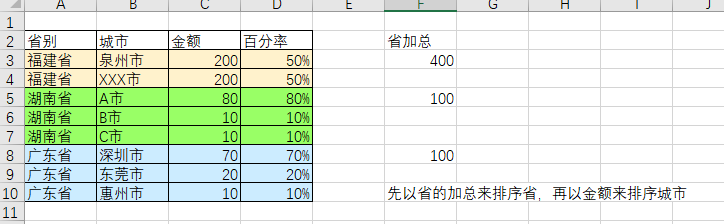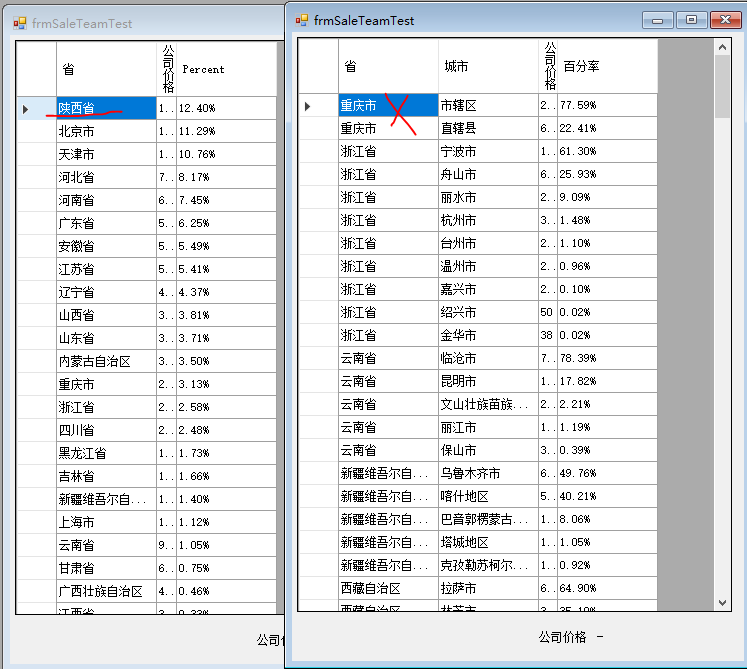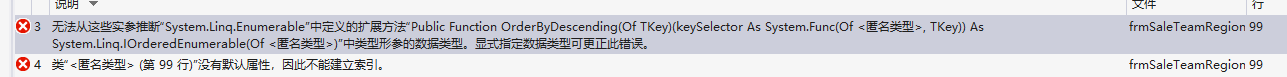vb.net 对datatable表格进行加工+加上百分率 5C2个回答

``````Dim query = dataTable.Rows.Cast(Of DataRow)().Select(Function(x) New With {.省别 = x("省别"), .城市 = x("城市"), 金额 = Val(x("金额")), .百分率 = Val(x("金额")) / dataTable.Rows.Cast(Of DataRow)().Where(Function(y) y("省别") = x("省别")).Select(Function(y) Val(y("金额"))).Sum() }).OrderBy(Function(x) x("省别")).ThenBy(Function(x) x("金额"))
For Each x In Query
MsgBox(x.省别 + x.城市 + " " + x.金额 + " " + x.百分率 * 100 + "%")
Next
``````
``````Dim query = dataTable.Rows.Cast(Of DataRow)().Select(Function(x) New With { .省别 = x("省别"), .城市 = x("城市"), 金额 = Val(x("金额")), .百分率 = Val(x("金额")) / dataTable.Rows.Cast(Of DataRow)().Where(Function(y) y("省别") = x("省别")).Select(Function(y) Val(y("金额"))).Sum() }).OrderBy(Function(x) x.省别).ThenBy(Function(x) x.金额)
``````weixin_40187983 是的，我把原本.OrderBy(Function(x) x("省别")).ThenBy(Function(x) x("金额")) 替代成下面你发的，但是一直有错误weixin_40187983 你是让我在去除后，改成这个吧？OrderBy(Function(x) dataTable.Rows.Cast(Of DataRow)().Where(Function(y) y("省别") = x("省别")).Select(Function(y) Val(y("金额"))).Sum())weixin_40187983 去除后，没有报错，就没有原本的排序而已weixin_40187983 好的，我等你weixin_40187983 贴好了weixin_40187983 我自己加下去，有报错不知道在那weixin_40187983 早上好，能帮我加到原本这句，成完整句吗？ query = dt.Rows.Cast(Of DataRow)().Select(Function(x) New With {.省 = x("省"), .城市 = x("城市"), .公司价格 = Val(x("公司价格")), .百分率 = Val(x("公司价格")) / dt.Rows.Cast(Of DataRow)().Where(Function(y) y("省") = x("省")).Select(Function(y) Val(y("公司价格"))).Sum()}).OrderByDescending(Function(x) x.省).ThenByDescending(Function(x) x.公司价格)weixin_40187983 回复操作员马善福（贵阳专业挖机）: 更正，我新附的图，左边单以省来排序， 右边是以省+城市来排序的，有没有办法让，右图的省份(以加总后的)，来排序省呢？然后右边的省排序就会和左边的一样了weixin_40187983 再请问一下，我新附的图，左边单以省来排序， 左边是以省+城市来排序的，有没有办法让，右图的省份(以加总后的)，来排序省呢？然后左边的省排序就会和左边的一样了weixin_40187983 你好，我将原本的表格，加工后。原本的表格(没有百分率的)，要如何移除呢？ 还有请问排序的话，order by desc 或是acs在这里的语法要怎么加呢？weixin_40187983 你好，我放到我的代码内后，有报错，请你帮我看一下！感谢